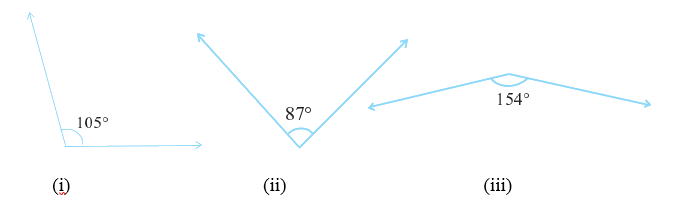# Ex.5.1 Q2 Lines and Angles Solution - NCERT Maths Class 7

Go back to  'Ex.5.1'

## Question

Find the supplement of each of the following angles:Video Solution
Lines & Angles
Ex 5.1 | Question 2

## Text Solution

What is known?

Measure of angles.

What is the unknown?
Measure of supplement of each angle.

Reasoning:
In a pair of angles, if the sum of the measures of the angles comes out to be $$180^\circ$$, angles are called supplementary angles. When two angles are supplementary, each angle is said to be the supplement of the other. If the given angle is $$x$$, then we can find the supplement by subtracting $$x$$ from $$180^\circ$$.

Steps:
(i) Given angle $$=105^\circ$$

Supplement angle of $$105^\circ$$

\begin{align} &= {180^\circ} - \text{given angle} \\ &= 180^\circ − 105^\circ \\ &= 75^\circ \end{align}

(ii) Given angle $$= 87^\circ$$

Supplement angle of $$87^\circ$$

\begin{align} &= 180^\circ -\text{given angle} \\ &= 180^\circ − 87^\circ \\ &= 93^\circ \end{align}

(iii) Given angle $$=154^\circ$$

Supplement angle of $$154^\circ$$

\begin{align} &= 180^\circ -\text{given angle} \\ &= 180^\circ − 154^\circ \\ &=26^\circ\end{align}

Learn from the best math teachers and top your exams

• Live one on one classroom and doubt clearing
• Practice worksheets in and after class for conceptual clarity
• Personalized curriculum to keep up with school